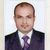# QlikView App Dev

Discussion Board for collaboration related to QlikView App Development.

cancel
Showing results for
Did you mean:Creator II

## Current YTD vs Previous year YTD

We are trying to compare current year YTD vs Prev year YTD.  Lets say we are in month of May 2014 so current year YTD would be Jan to May 2014 revenue. And we need to compare this with previous yr YTD revenue which should be Jan to May 2013 so how can we write and expression so that the prev year YTD will calculate only these 5 months  Jan to May 2013 and exclude rest while calculating revenue?

4 RepliesMVP

Several ways are there. A better one : Year Over Year ComparisonMVP

Write like

For Current YTD

=SUM({\$<YourDateField = {'>=\$(=YearStart(Today()))<=\$(=MonthEnd(Today()))'}>}Sales)

For Previous YTD

=SUM({\$<YoureDateField = {'>=\$(=AddMonths( YearStart (Today()),-12))<=\$(=AddMonths( MonthEnd (Today()),-12))'}>}Sales)Partner - Creator III

Current Year- Sum({<Date={">=YearStart(Today())<=\$(=Today())"}>}Sales)

Previous Year- Sum({<Date={">=\$(=YearStart(\$(=MakeDate(Year(Today())-1,Month(Today()),Day(Today())))))<=\$(=MakeDate(Year(Today())-1,Month(Today()),Day(Today())))"}>}Sales)Not applicable

Hello @Anand Chouhan

I used your code:

=Count({\$<BookingDate = {'>=\$(=YearStart(Today()))<=\$(=MonthEnd(Today()))'}>}AssignmentNumber)

=Count({\$<BookingDate = {'>=\$(=AddMonths( YearStart (Today()),-12))<=\$(=AddMonths( MonthEnd (Today()),-12))'}>}AssignmentNumber)

It works perfectly.

Then I wanted to add another preceding year.. Following same form.. but this did not work at all.. Any idea how to make this work?

=Count({\$<BookingDate = {'>=\$(=AddMonths( YearStart (Today()),-24))<=\$(=AddMonths( MonthEnd (Today()),-24))’}>}AssignmentNumber)

Thanks

/Oskar